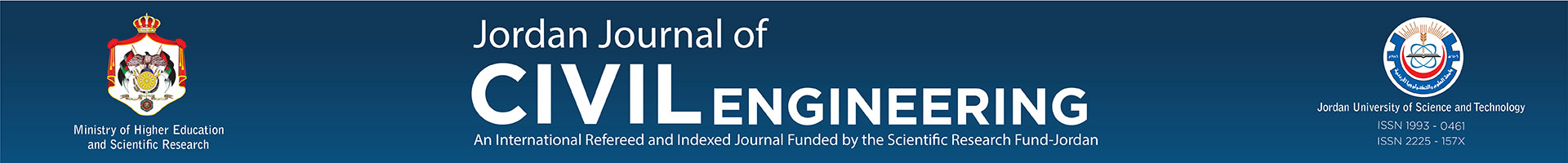AN OPEN ACCESS JOURNAL
JJCE Submission Home

Exponential Model For Predicting The Sorption Isotherms-evaluation, Statistical Appraisal And Comparison With Existing Models

 Submitted 2009-08-30 Last Update 2009-08-30 Title Exponential Model For Predicting The Sorption Isotherms-evaluation, Statistical Appraisal And Comparison With Existing Models Author(s) Author #1Author title:Name: Waddah Salman AbdullahOrg: Associate Professor of Civil Engineering, Faculty of Engineering, Jordan University of Science and Technology, Irbid, Jordan.Country: Email: waddah@just.edu.jo Other Author(s) Contact Author Author #1Alt Email: waddah@just.edu.joTelephone: Keywords Geo-environment, Diffusion, Sorption isotherm, Modeling, Freundlich model, Langmuir model, Statistical appraisal, Goodness-of-fit. Abstract A nonlinear model simulating the relationship between the mass of solute sorbed on clay mineral surfaces and in equilibrium with the concentration of solute in the pore fluid has been discussed in this work. The model and the procedure for obtaining the parameter (β) associated with the model were presented. The predicted cadmium isotherms for two types of clayey soils using the exponential model were in excellent agreement with experimental results (Shackelford and Daniel, 1991). Sorption isotherm predicted by the exponential model was compared with other existing models such as Freundlich model and Langmuir model. Seven statistical goodnessof-fit measures were used to evaluate prediction accuracy of the exponential model as well as the Langmuir model and the Freundlich model. All seven statistical goodness-of-fit measures indicated that the exponential model has the best prediction accuracy among the sorption isotherm prediction models. For instance, the error norm for the exponential model was Ne = 0.15 (best value of Ne is zero) for the kaolinite clay, while Ne = 2.95 and Ne = 6.1 for Langmuir model and Freundlich model, respectively. Furthermore, for Lufkin clay, the error norm for the exponential model was Ne = 1.6, while Ne = 264.4 and Ne = 15.1 for Langmuir model and Freundlich model, respectively. The modeling efficiency (EF), Nash-Sutcliffe coefficient-of-efficiency (E) and the index-of-agreement (d) values (best value for EF, E and d is one for the three measures) are best for the exponential model and for the two considered soils. For example, EF = 0.992, E = 0.999, and d = 0.999 for the exponential model (Lufkin clay). However, EF = -0.71, E = -20.5 and d = 0.07 for Langmuir model, and EF = 0.79, E = 0.93 and d = 0.95 for Freundlich model for the same clay. Paper88.pdf (240KB)